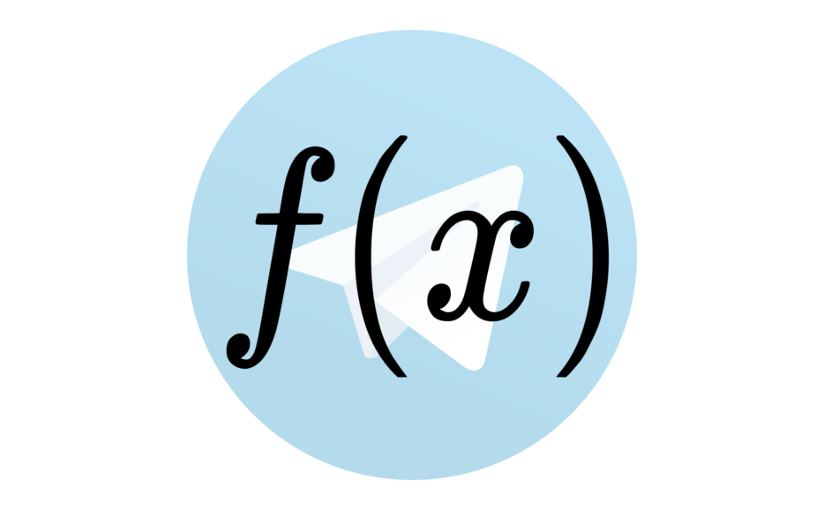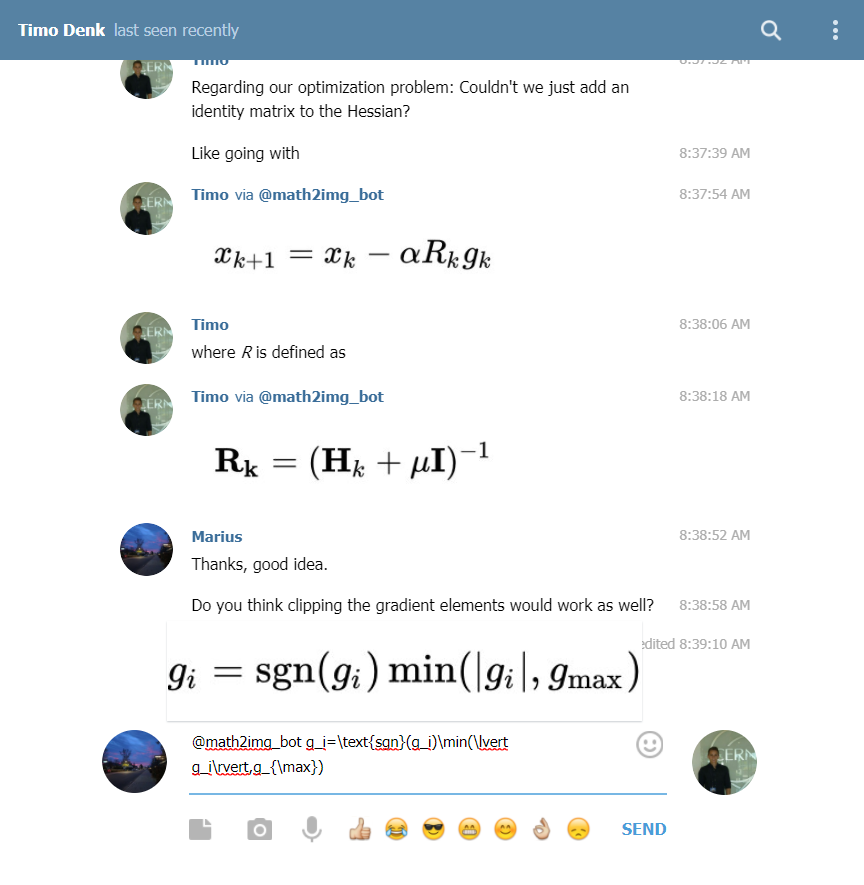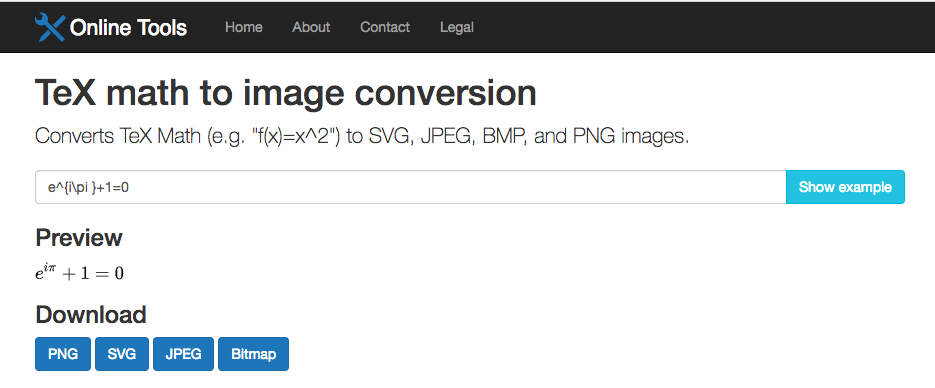# TeX Math to Image Conversion

· Timo Denk

This post functions as a quick development update on the Math to Image Conversion Bot (on Telegram), the TeX math to image conversion tool (at tools.timodenk.com), and the API that serves them both. The objective is to convert TeX math-code into images.

For example, the expression \sum _{n=0}^{\infty }{\frac {f^{(n)}(a)}{n!}}\,(x-a)^{n} yields this image:This page on StackExchange gives a good overview about the math-expressions which can be used.

Both, the bot and the online tool, are using the same API. Source code and specification of which can be found on GitHub /Simsso/TeX2img-API.

The Telegram inline-bot let’s users send mathematical statements to their chat partner. Type @math2img_bot into the input field, followed by the mathematical expression, to send rendered math images. Also, users can text the bot in a private chat and send expression or ask for examples with the /sample command. The source code is available on Github /Simsso/Telegram-Math2Image-Bot. An example of usage is shown in the screenshot below.The new online tool /tex-math-to-image-conversion simply converts a given expression into an image which can then be downloaded by the user. Supported file formats are SVG, PNG, BMP, and JPEG. This is what it looks like: